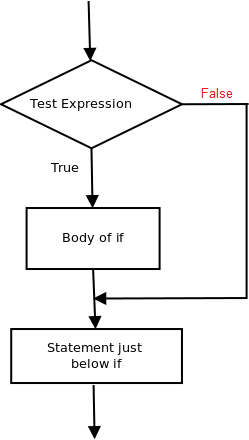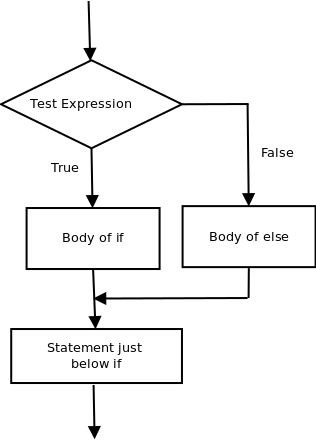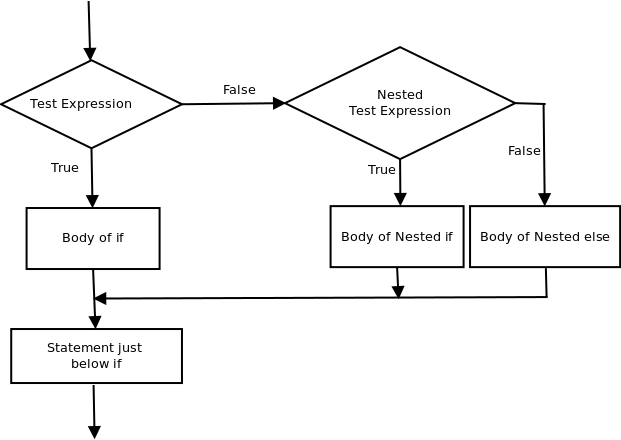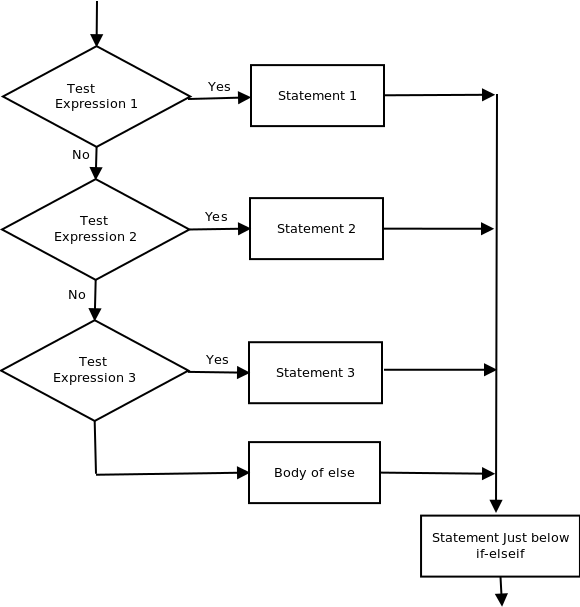# Decision Making in PL/SQL (if-then , if-then-else, Nested if-then, if-then-elsif-then-else )

There come situations in real life when we need to make some decisions and based on these decisions, we decide what should we do next. Similar situations arise in programming also where we need to make some decisions and based on these decisions we will execute the next block of code.

Decision-making statements in programming languages decide the direction of flow of program execution. Decision-making statements available in pl/SQL are:

1. if then statement
2. if then else statements
3. nested if-then statements

1. if then statement
if then statement is the most simple decision-making statement. It is used to decide whether a certain statement or block of statements will be executed or not i.e if a certain condition is true then a block of statement is executed otherwise not.

Syntax:

```if condition then
-- do something
end if;
```

Here, condition after evaluation will be either true or false. if statement accepts boolean values – if the value is true then it will execute the block of statements below it otherwise not.
if and endif consider as a block here.

Example:-

 `declare` `-- declare the values here ` ` `  `begin` ` `  `if condition ``then` `dbms_output.put_line(``'output'``); ` ` `  `end` `if; ` ` `  `dbms_output.put_line(``'output2'``); ` `end``; ``-- pl/sql program to illustrate If statement ` `declare` `num1 number:= 10; ` `num2 number:= 20; ` ` `  `begin` ` `  `if num1 > num2 ``then` `dbms_output.put_line(``'num1 small'``); ` `end` `if; ` ` `  `dbms_output.put_line(``'I am Not in if'``); ` ` `  `end``; `

As the condition present in the if statement is false. So, the block below the if statement is not executed.
Output:

```I am Not in if
```
2. if – then- else:
The if statement alone tells us that if a condition is true it will execute a block of statements and if the condition is false it won’t. But what if we want to do something else if the condition is false. Here comes the else statement. We can use the else statement with if statement to execute a block of code when the condition is false.
Syntax:-

```if (condition) then
-- Executes this block if
-- condition is true
else
-- Executes this block if
-- condition is false
```Example:-

 `-- pl/sql program to illustrate If else statement ` `declare` `num1 number:= 10; ` `num2 number:= 20; ` ` `  `begin` ` `  `if num1 < num2 ``then` `dbms_output.put_line(``'i am in if block'``); ` ` `  `ELSE` `dbms_output.put_line(``'i am in else Block'``); ` `end` `if; ` ` `  `dbms_output.put_line(``'i am not in if or else Block'``); ` `end``; `

Output:-

```i'm in if Block
i'm not in if and not in else Block
```

The block of code following the else statement is executed as the condition present in the if statement is false after calling the statement which is not in block(without spaces).

3. nested-if-then:
A nested if-then is an if statement that is the target of another if statement. Nested if-then statements mean an if statement inside another if statement. Yes, PL/SQL allows us to nest if statements within if-then statements. i.e, we can place an if then statement inside another if then statement.

Syntax:-

```if (condition1) then
-- Executes when condition1 is true
if (condition2) then
-- Executes when condition2 is true
end if;
end if;
````-- pl/sql program to illustrate nested If statement ` `declare` `num1 number:= 10; ` `num2 number:= 20; ` `num3 number:= 20; ` ` `  `begin` `if num1 < num2 ``then` `dbms_output.put_line(``'num1 small num2'``); ` ` `  `  ``if num1 < num3 ``then`   `  ``dbms_output.put_line(``'num1 small num3 also'``); ` `  ``end` `if; ` ` `  `end` `if; ` ` `  `dbms_output.put_line(``'after end if'``); ` `end``; `

Output:-

```num1 small num2
num1 small num3 also
after end if
```
Here, a user can decide among multiple options. The if then statements are executed from the top down. As soon as one of the conditions controlling the if is true, the statement associated with that if is executed, and the rest of the ladder is bypassed. If none of the conditions is true, then the final else statement will be executed.

Syntax:-

```if (condition) then
--statement
elsif (condition) then
--statement
.
.
else
--statement
endif
```

Flow Chart:-Example:-

 `-- pl/sql program to illustrate if-then-elif-then-else ladder ` `declare` `num1 number:= 10; ` `num2 number:= 20; ` ` `  `begin` ` `  `if num1 < num2 ``then` `dbms_output.put_line(``'num1 small'``); ` ` `  `ELSIF num1 = num2 ``then` `dbms_output.put_line(``'both equal'``); ` ` `  `ELSE` `dbms_output.put_line(``'num2 greater'``); ` `end` `if; ` ` `  `dbms_output.put_line(``'after end if'``); ` `end``; `

Output:-

```num1 small
after end if
```

Attention reader! Don’t stop learning now. Get hold of all the important CS Theory concepts for SDE interviews with the CS Theory Course at a student-friendly price and become industry ready.

My Personal Notes arrow_drop_upCheck out this Author's contributed articles.

If you like GeeksforGeeks and would like to contribute, you can also write an article using contribute.geeksforgeeks.org or mail your article to contribute@geeksforgeeks.org. See your article appearing on the GeeksforGeeks main page and help other Geeks.

Please Improve this article if you find anything incorrect by clicking on the "Improve Article" button below.

Improved By : Akanksha_Rai

Article Tags :
Practice Tags :

1

Please write to us at contribute@geeksforgeeks.org to report any issue with the above content.